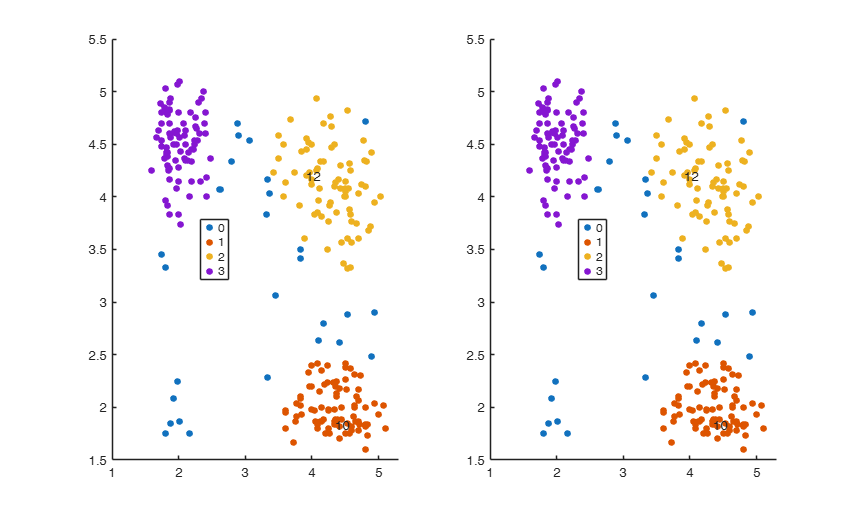# ClusterRelabel

ClusterRelabel enables to control the labels of the clusters which contain predefined units

## Syntax

• IDXrelabelled=ClusterRelabel(IDX,pivotunits)example
• [IDXrelabelled, idxMapping]=ClusterRelabel(___)example

## Description

 IDXrelabelled =ClusterRelabel(IDX, pivotunits) Start with labelling produced by tclustIC and produce consistent labels.

 [IDXrelabelled, idxMapping] =ClusterRelabel(___) Example with detailed description of output element OldAndNewIndexes.

## Examples

expand all

Y=load('geyser2.txt');
% A small number of subsamples just to show whow the procedure works.
nsamp=10;
out=tclustIC(Y,'cleanpool',false,'plots',1,'nsamp',10,'whichIC','CLACLA');
% Make sure that units [23 54] are whenever possible respectively in
% cluster 1 and 2
UnitsSameGroup=[23 54];
IDXCLAnew=ClusterRelabel(out.IDXCLA,UnitsSameGroup);

###Example with detailed description of output element OldAndNewIndexes.

Random seed to be example ro replicate the results.

rng(1000)
k=3;
[out]=tclust(Y,k,0.10,10);
% Make sure that group which contains
% unit 10 is always labelled with number 1. Similarly,
% make sure that the group which contains unit 12 is always labelled
% with number 2,
UnitsSameGroup=[10;12];
[idxnew, OldNewIndexes]=ClusterRelabel({out.idx}, UnitsSameGroup);
% In this case OldNewIndexes is equal to
% 3 1
% 3 2
% It means that in the first iteration labels 1 and 3 have swapped
% while in the second iteration label 3 and 2 have swapped
subplot(1,2,1)
gscatter(Y(:,1),Y(:,2),out.idx)
text(Y(UnitsSameGroup,1),Y(UnitsSameGroup,2),num2str(UnitsSameGroup))
subplot(1,2,2)
gscatter(Y(:,1),Y(:,2),idxnew{:})
text(Y(UnitsSameGroup,1),Y(UnitsSameGroup,2),num2str(UnitsSameGroup))
% Now (as is evident from the right panel) unit which contains group 10
% has label '1' while group which contains unit 12 has label '2'.
ClaLik with untrimmed units selected using crisp criterion
Total estimated time to complete tclust:  0.94 seconds## Input Arguments

### IDX — Assignment of units to groups for different values of c (restriction factor) and k (number of groups). Cell.

Cell of size length(kk)-times length(cc), where kk is the vector which contains the number of groups which have been considered and cc is the vector which contains the values of the restriction factor. Each element of the cell is a vector of length n containing the assignment number of each unit using a particular classification model.

Data Types:  cell

### pivotunits — list of the units which must (whenever possible) have the same label. Numeric vector.

For example if pivotunits=[20 26], means that group which contains unit 20 is always labelled with number 1. Similarly, the group which contains unit 26 is always labelled with number 2, (unless it is found that unit 26 already belongs to group 1). In general, group which contains unit UnitsSameGroup(r) where r=2, ...length(kk)-1 is labelled with number r (unless it is found that unit UnitsSameGroup(r) has already been assigned to groups 1, 2, ..., r-1).

Data Types:  integer vector

## Output Arguments

### IDXrelabelled —cell with the same size as input cell IDX and with the same meaning of input cell IDX but with consistent labels. Cell

Group which contains unit UnitsSameGroup(1) is labelled with number 1. In general. Group which contains UnitsSameGroup(r) where r=2, ...length(kk)-1 is labelled with number r (unless it is found that unit UnitsSameGroup(r) has already been assigned to groups 1, 2, ..., r-1).

### idxMapping —indexes of the permutations associated with IDX{1,1}.  r-by-2 matrix

Matrix of size r-by-2 which keeps track of all the permutations which have been done. For example if idxMapping is equal to [3, 1; 3, 2], it means that in the first iteration labels 1 and 3 have swapped, while in the second iteration label 3 and 2 have swapped. If no swapping was necessary idxMapping is empty.

Cerioli, A., Garcia-Escudero, L.A., Mayo-Iscar, A. and Riani M. (2017), Finding the Number of Groups in Model-Based Clustering via Constrained Likelihoods, "Journal of Computational and Graphical Statistics", pp. 404-416, https://doi.org/10.1080/10618600.2017.1390469